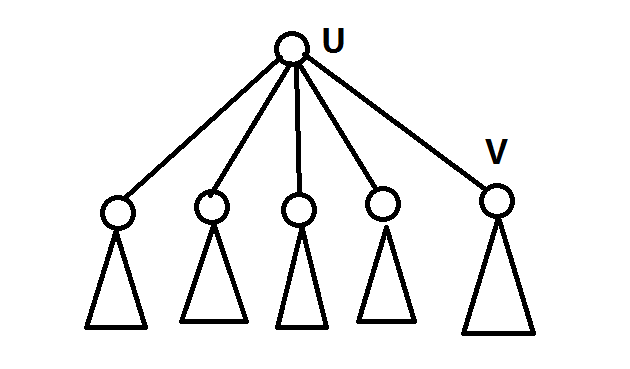点分治学习笔记

原理（基于重心的分割）$ps.$重心有一个比较神奇的性质：每一个子树的大小都不超过n/2

关于重心性质的证明求解重心的代码

void getroot(int u,int fa){
sz[u]=1; mxs[u]=0;//sz数组是子树的节点个数，mxs是maxson的缩写，表示的是最大的子树的节点数
for(int e=H[u];e;e=E[e].nt){//前向星的遍历方式
int v=E[e].to;
if (v==fa) continue;//如果遇到了父亲那么就跳过
getroot(v,u);//向下递归求解
sz[u]+=sz[v];
mxs[u]=max(mxs[u],sz[v]);//计算mxs
}
mxs[u]=max(mxs[u],sum-sz[u]);//这是另外一半的树，这也是这棵树的子树（整棵树被当作了无根树）
if(mxs[u]<mxs[rt]||rt==0) rt=u;//判断是不是重心
}

“分而治之”的“治”操作

void divide
计算当前全局的答案
记录访问节点
枚举子节点{
如果访问过就跳过
将局部重复解减去
递归搜索子树重心
}

void solve(int u){
calc(u,1,0);//计算答案
vis[u]=1;//标记已经访问过了
for(int e=H[u];e;e=E[e].nt){
int v=E[e].to;
if(vis[v]) continue;//如果已经访问过了就不用访问了，也起到了不算父亲的情况
calc(v,0,E[e].w);//容斥原理减去所有重复的状态
MX=inf; sum=sz[v]; rt=0; getroot(v,-1);//算出子树的重心
solve(rt);//递归求解
}
}$Chhokmah$（叹了一口气）：终于差不多讲完了，接下来就是题目。。。

习题

洛谷模板3806

ac代码

#include<bits/stdc++.h>
#define N 100005
#define inf 0x3f3f3f3f
using namespace std;
struct edge{
int to,nt,w;
}E[N<<1];
int H[N],ans,sz[N],mxs[N],S,vis[N],dis[N];
int n,m,sum,cnt,tot,rt,MX;
int w=0,x=0;char ch=0;
while(!isdigit(ch))w|=ch=='-',ch=getchar();
while(isdigit(ch))x=(x<<1)+(x<<3)+(ch^48),ch=getchar();
return w?-x:x;
}
void addedge(int u,int v,int w){
E[++cnt]=(edge){v,H[u],w}; H[u]=cnt;
}
void getroot(int u,int fa){
sz[u]=1; mxs[u]=0;
for(int e=H[u];e;e=E[e].nt){
int v=E[e].to;
if (v==fa||vis[v]) continue;
getroot(v,u);
sz[u]+=sz[v];
mxs[u]=max(mxs[u],sz[v]);
}
mxs[u]=max(mxs[u],sum-sz[u]);
if(mxs[u]<MX) rt=u,MX=mxs[u];
}
void getdis(int u,int fa,int dist){
dis[++tot]=dist;
for(int e=H[u];e;e=E[e].nt){
int v=E[e].to;
if(v==fa||vis[v]) continue;
getdis(v,u,dist+E[e].w);
}
}
void calc(int u,int fg,int len){
tot=0;
getdis(u,-1,len);
for(int i=1;i<tot;i++){
for(int j=i+1;j<=tot;j++){
if(fg) ans[dis[i]+dis[j]]++;
else ans[dis[i]+dis[j]]--;
}
}
}
void solve(int u){
calc(u,1,0);
vis[u]=1;
for(int e=H[u];e;e=E[e].nt){
int v=E[e].to;
if(vis[v]) continue;
calc(v,0,E[e].w);
MX=inf; sum=sz[v]; rt=0; getroot(v,-1);
solve(rt);
}
}
int main(){
for(int i=1;i<n;i++){
}
sum=n; MX=inf;
getroot(1,-1);
solve(rt);
while(m--){
if(ans[k]>0) printf("AYE\n"); else printf("NAY\n");
}
return 0;
}

[poj1741]Tree

题目描述

Give a tree with n vertices,each edge has a length(positive integer less than 1001).
Define dist(u,v)=The min distance between node u and v.
Give an integer k,for every pair (u,v) of vertices is called valid if and only if dist(u,v) not exceed k.
Write a program that will count how many pairs which are valid for a given tree.

解法

$ps.$不要试图提交我的代码，会让你出锅的。QAQ（我写的洛谷上的程序，懒得改了）。

ac代码

# include <cstdio>
# include <cstring>
# include <algorithm>
# include <ctype.h>
# include <iostream>
# include <cmath>
# include <map>
# include <vector>
# include <queue>
# define LL long long
# define ms(a,b) memset(a,b,sizeof(a))
# define ri (register int)
# define inf (0x7f7f7f7f)
# define pb push_back
# define fi first
# define se second
# define pii pair<int,int>
# define File(s) freopen(s".in","r",stdin),freopen(s".out","w",stdout)
using namespace std;
inline int gi(){
int w=0,x=0;char ch=0;
while(!isdigit(ch)) w|=ch=='-',ch=getchar();
while(isdigit(ch)) x=(x<<1)+(x<<3)+(ch^48),ch=getchar();
return w?-x:x;
}
# define N 40005
struct edge{
int to,nt,w;
}E[N<<1];
int cnt,rt,sum,n,k;
LL ans;
int H[N],sz[N],mxs[N],dis[N],d[N],mx;
bool vis[N];
void addedge(int u,int v,int w){
E[++cnt]=(edge){v,H[u],w}; H[u]=cnt;
}
void get_root(int u,int fa){
sz[u]=1; mxs[u]=0;
for (int e=H[u];e;e=E[e].nt){
int v=E[e].to;
if (v==fa||vis[v]) continue;
get_root(v,u);
sz[u]+=sz[v];
mxs[u]=max(mxs[u],sz[v]);
}
mxs[u]=max(mxs[u],sum-sz[u]);
if (mxs[u]<mx) rt=u,mx=mxs[u];
}
void get_deep(int u,int fa){
dis[++cnt]=d[u];
for (int e=H[u];e;e=E[e].nt){
int v=E[e].to;
if (v==fa||vis[v]) continue;
d[v]=d[u]+E[e].w;
get_deep(v,u);
}
}
LL calc(int u,int now){
cnt=0; d[u]=now; get_deep(u,-1);
sort(dis+1,dis+cnt+1);
int l=1,r=cnt; LL res=0;
while (l<r){
if (dis[l]+dis[r]<=k) res+=r-l,l++;
else r--;
}
return res;
}
void divide(int u){
ans+=calc(u,0);
vis[u]=1;
for (int e=H[u];e;e=E[e].nt){
int v=E[e].to;
if (vis[v]) continue;
ans-=calc(v,E[e].w);
sum=sz[v]; rt=-1; mx=inf;
get_root(v,-1);
divide(rt);
}
}
int main(){
ms(vis,0); n=gi();
for (int i=1;i<n;i++){
int u=gi(),v=gi(),w=gi();